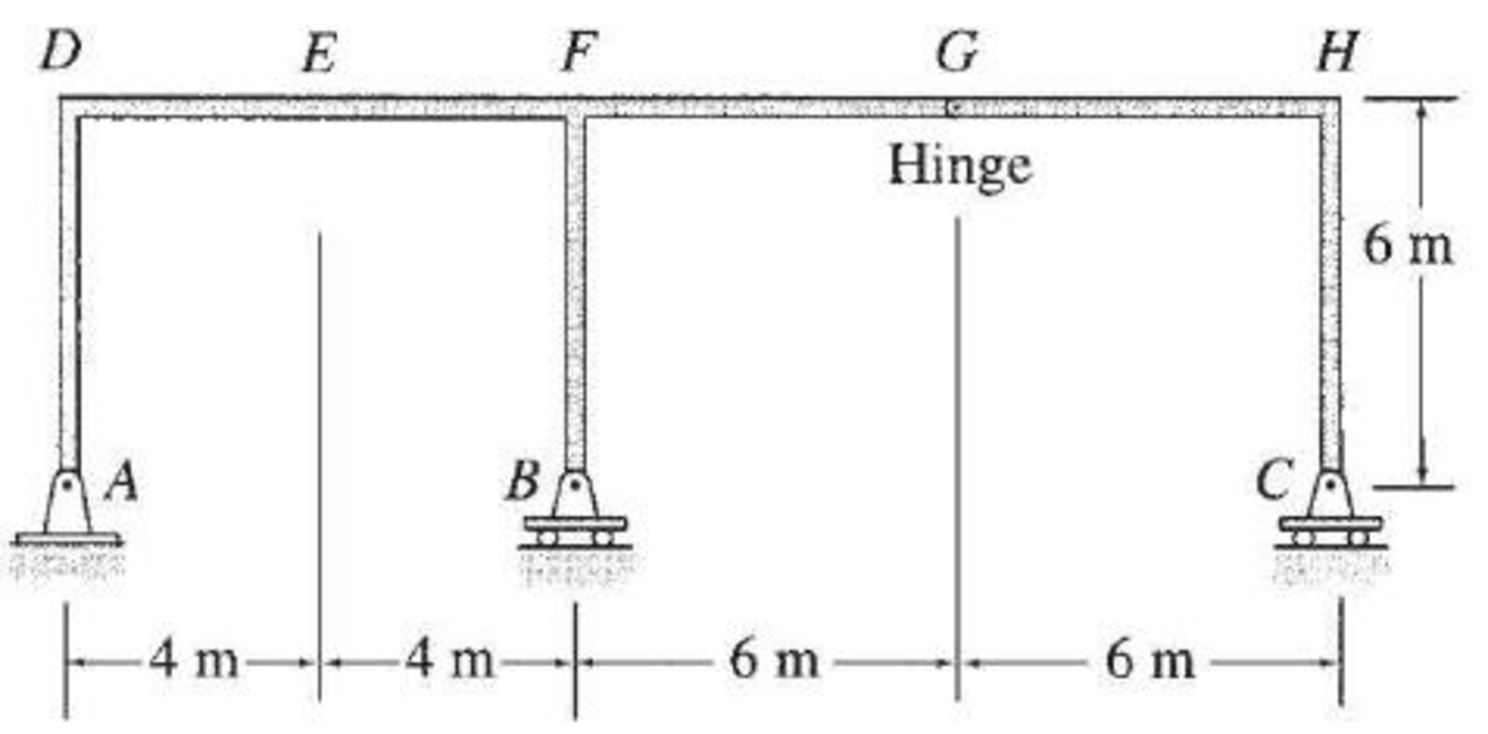# Draw the influence lines for the vertical reactions at supports A , B , C, and the shear and bending moment at point E of the frame shown in Fig. P8.39. FIG. PB.39

#### Solutions

Chapter
Section
Chapter 8, Problem 39P
Textbook Problem
125 views

## Draw the influence lines for the vertical reactions at supports A, B, C, and the shear and bending moment at point E of the frame shown in Fig. P8.39.FIG. PB.39

To determine

Draw the influence lines for the vertical reactions at supports A, B, C and the shear and bending moment at point E.

### Explanation of Solution

Calculation:

Apply a 1 kN unit moving load at a distance of x from left end D.

Sketch the free body diagram of frame as shown in Figure 1.

Influence line for vertical reaction at supports C.

Refer Figure 1.

Find the equation of vertical reaction at supports C.

Apply 1 kN load just left of G (0x14m).

Consider section GH.

Take moment at G from C.

Consider clockwise moment as positive and anticlockwise moment as negative.

ΣMG=0Cy(6)=0Cy=0

Apply 1 kN load just right of G (14mx20m).

Consider section GH.

Take moment at G from C.

Consider clockwise moment as positive and anticlockwise moment as negative.

ΣMG=0Cy(6)+1(x14)=06Cy=x14Cy=x673

Thus, the equation of vertical reaction at supports C as follows,

Cy=0, (0x14m)        (1)

Cy=x673, (14mx20m)        (2)

Find the influence line ordinate of Cy at H using Equation (2).

Substitute 20 m for x in Equation (2).

Cy=20673=10373=33=1kN

Thus, the influence line ordinate of Cy at H is 1kN/kN.

Similarly calculate the influence line ordinate of Cy at various points on the frame and summarize the values in Table 1.

 x (m) Points Influence line ordinate of Cy(kN/kN) 0 D 0 4 E 0 8 F 0 14 G 0 20 H 1

Sketch the influence line diagram for vertical reaction at supports C using Table 1 as shown in Figure 2.

Influence line for vertical reaction at support A.

Apply 1 kN load just left of F (0x8m).

Refer Figure 1.

Find the equation of vertical reaction at supports C.

Consider section DF.

Take moment at B from A.

Consider clockwise moment as positive and anticlockwise moment as negative.

ΣMB=0Ay(8)1(8x)=08Ay=8xAy=1x8

Apply 1 kN load just right of F.

Consider section FH.

Consider moment at B from A is equal to from C.

Consider clockwise moment as positive and anticlockwise moment as negative.

ΣMB=0Ay(8)=Cy(12)1(x8)8Ay=12Cyx+8        (3)

Find the equation of vertical reaction at A from F to G (8mx14m).

Substitute 0 for Cy in Equation (3).

8Ay=12(0)x+88Ay=8xAy=1x8

Find the equation of vertical reaction at A from G to H (14mx20m).

Substitute x673 for Cy in Equation (3).

8Ay=12(x673)x+8=2x28x+8=x20Ay=x852

Thus, the equation of vertical reaction at supports A as follows,

Ay=1x8, (0x14m)        (4)

Ay=x852, (14mx20m)        (5)

Find the influence line ordinate of Ay at G using Equation (4).

Substitute 14 m for x in Equation (4).

Ay=1148=0.75kN

Thus, the influence line ordinate of Ay at G is 0.75kN/kN.

Similarly calculate the influence line ordinate of Ay at various points on the frame and summarize the values in Table 2.

 x (m) Points Influence line ordinate of Ay(kN/kN) 0 D 1 4 E 0.5 8 F 0 14 G −0.75 20 H 0

Sketch the influence line diagram for the vertical reaction at support A using Table 2 as shown in Figure 3.

Influence line for vertical reaction at support B.

Apply a 1 kN unit moving load at a distance of x from left end C.

Refer Figure 1.

Apply vertical equilibrium in the system.

Consider upward force as positive and downward force as negative.

Ay+By+Cy1=0By=1AyCy        (6)

Find the equation of vertical support reaction (By) from D to G (0x14m) using Equation (6).

Substitute 1x8 for Ay and 0 for Cy in Equation (6).

By=1(1x8)0=11+x8=x8

Find the equation of vertical support reaction (By) from G to H (14mx20m) using Equation (6).

Substitute x852 for Ay and x673 for Cy in Equation (6).

By=1(x852)(x673)=1x8+52x6+73=3567x24

Thus, the equation of vertical support reaction at B as follows,

By=x8, (0x14m)        (7)

By=3567x24, (14mx20m)        (8)

Find the influence line ordinate of By at F using Equation (7).

Substitute 8 m for x in Equation (7).

By=88=1kN

Thus, the influence line ordinate of By at F is 1kN/kN

### Still sussing out bartleby?

Check out a sample textbook solution.

See a sample solution

#### The Solution to Your Study Problems

Bartleby provides explanations to thousands of textbook problems written by our experts, many with advanced degrees!

Get Started

Find more solutions based on key concepts
Using Excel or a spreadsheet of your choice, create a degrees Fahrenheit to degrees Celsius conversion table fo...

Engineering Fundamentals: An Introduction to Engineering (MindTap Course List)

In addition to the reasons mentioned in this exercise, why else might you want to obtain a copy of yourcredit r...

Enhanced Discovering Computers 2017 (Shelly Cashman Series) (MindTap Course List)

What are the elements of a business impact analysis?

Principles of Information Security (MindTap Course List)

What is a solid state storage device?

Principles of Information Systems (MindTap Course List)

What can cause scratches on the surface of a workpiece?

Precision Machining Technology (MindTap Course List)

What can cause a properly sized electrode holder to overheat?

Welding: Principles and Applications (MindTap Course List)

If your motherboard supports ECC DDR3 memory, can you substitute non-ECC DDR3 memory?

A+ Guide to Hardware (Standalone Book) (MindTap Course List)